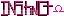-=+=- -=+=- -=+=- -=+=- -=+=- -=+=- -=+=- -=+=- -=+=- -=+=- -=+=- -=+=- -=+=- -=+=- -=+=- -=+=- -=+=- -=+=- -=+=- -=+=- -=+=- -=+=- -=+=- -=+=- -=+=- -=+=- -=+=- -=+=- -=+=- -=+=- (c) WidthPadding Industries 1987 0|712|0 -=+=- -=+=- -=+=- -=+=- -=+=- -=+=- -=+=- -=+=- -=+=- -=+=- -=+=- -=+=- -=+=- -=+=- -=+=- -=+=- -=+=- -=+=- -=+=- -=+=- -=+=- -=+=- -=+=- -=+=- -=+=- -=+=- -=+=- -=+=- -=+=- -=+=-
SoCoder -> Article Home -> Advanced Techniques

SchererererCreated : 29 January 2009

### Logic (part 1)

Part of the Series on Discrete Structures

Thursday, 29 January 2009, 12:31
PhoenixThursday, 29 January 2009, 20:42
SchererererGreat job Phoenix!

> Reveal 🔎Thursday, 29 January 2009, 21:26
blanko1324Good article; a great refresher from my high school logic unit. Can't wait for more.